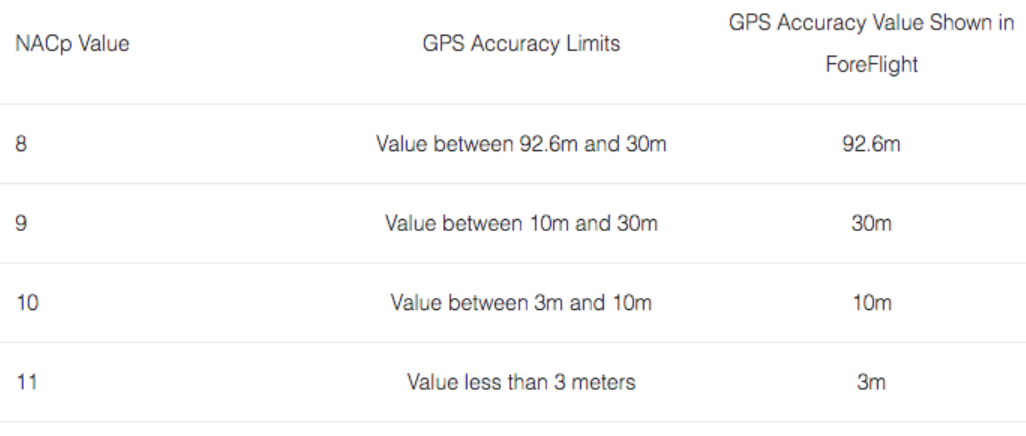# Why does my DUAL XGPS170 show a GPS accuracy of 30m?

The DUAL Status app sends ForeFlight the GPS accuracy value and it uses the aviation standard of Navigational Accuracy Category for Position (NACp). The NACp level is determined by Estimate Position Uncertainty (EPU). EPU is an accuracy bound on the horizontal position, which is defined as the radius of a circle with its center on the reported position, as such the probability of the actual position lying within the circle is 95%.

If the DUAL Status app determines that your position information has a NACp value of 9, then ForeFlight will show an accuracy of 30m. The accuracy is actually somewhere between 10m and 30m.

If the NACp value is calculated as being a 10, then ForeFlight will show an accuracy of 10m and the actual accuracy will be between 3m and 10m.

Here is a table that describes the NACp values and calculated accuracy on the NACp scale.Image 1. NACp values and the corresponding accuracy value that is shown in ForeFlight.